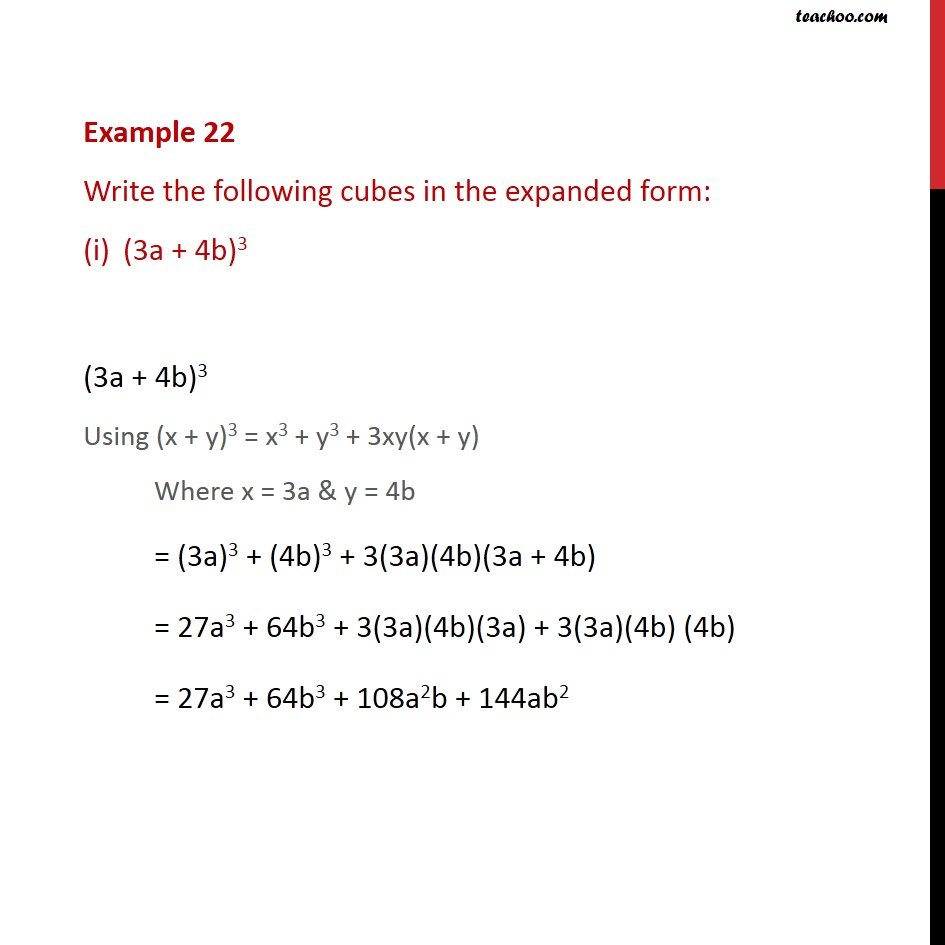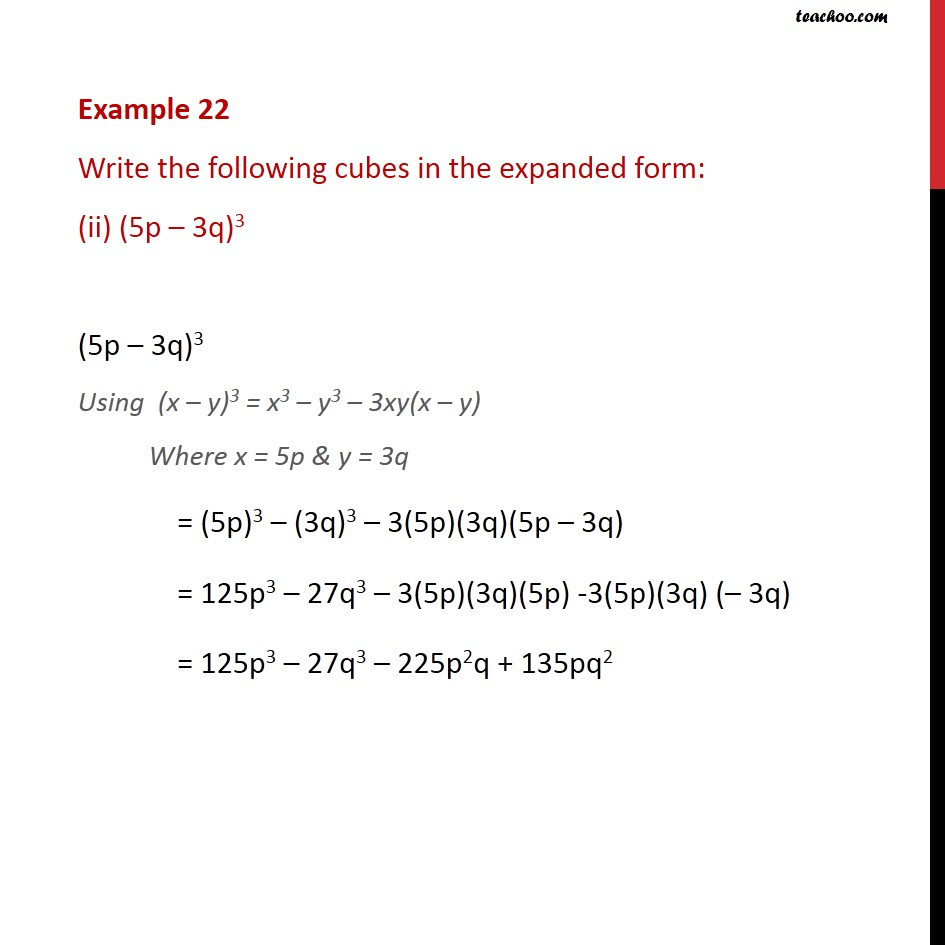Learn all Concepts of Polynomials Class 9 (with VIDEOS). Check - Polynomials Class 91. Chapter 2 Class 9 Polynomials
2. Serial order wise
3. Examples

Transcript

Example 22 Write the following cubes in the expanded form: (3a + 4b)3 (3a + 4b)3 Using (x + y)3 = x3 + y3 + 3xy(x + y) Where x = 3a & y = 4b = (3a)3 + (4b)3 + 3(3a)(4b)(3a + 4b) = 27a3 + 64b3 + 3(3a)(4b)(3a) + 3(3a)(4b) (4b) = 27a3 + 64b3 + 108a2b + 144ab2 Example 22 Write the following cubes in the expanded form: (ii) (5p – 3q)3 (5p – 3q)3 Using (x – y)3 = x3 – y3 – 3xy(x – y) Where x = 5p & y = 3q = (5p)3 – (3q)3 – 3(5p)(3q)(5p – 3q) = 125p3 – 27q3 – 3(5p)(3q)(5p) -3(5p)(3q) (– 3q) = 125p3 – 27q3 – 225p2q + 135pq2

Examples

Chapter 2 Class 9 Polynomials
Serial order wise